LORENE
Lorene::Eos_incomp Class Reference

Equation of state of incompressible matter (relativistic case). More...

#include <eos.h>

Inheritance diagram for Lorene::Eos_incomp: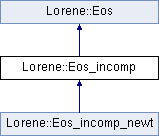## Public Member Functions

Eos_incomp (double rho_c)
Standard constructor. More...

Eos_incomp (double rho_c, double ent_c)
Standard constructor with log-enthalpy threshold. More...

Eos_incomp (const Eos_incomp &)
Copy constructor. More...

virtual ~Eos_incomp ()
Destructor. More...

void operator= (const Eos_incomp &)
Assignment to another Eos_incomp. More...

virtual bool operator== (const Eos &) const
Comparison operator (egality) More...

virtual bool operator!= (const Eos &) const
Comparison operator (difference) More...

virtual int identify () const
Returns a number to identify the sub-classe of Eos the object belongs to. More...

virtual void sauve (FILE *) const
Save in a file. More...

virtual double nbar_ent_p (double ent, const Param *par=0x0) const
Computes the baryon density from the log-enthalpy. More...

virtual double ener_ent_p (double ent, const Param *par=0x0) const
Computes the total energy density from the log-enthalpy. More...

virtual double press_ent_p (double ent, const Param *par=0x0) const
Computes the pressure from the log-enthalpy. More...

virtual double der_nbar_ent_p (double ent, const Param *par=0x0) const
Computes the logarithmic derivative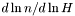from the log-enthalpy. More...

virtual double der_ener_ent_p (double ent, const Param *par=0x0) const
Computes the logarithmic derivative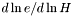from the log-enthalpy. More...

virtual double der_press_ent_p (double ent, const Param *par=0x0) const
Computes the logarithmic derivative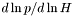from the log-enthalpy. More...

virtual double csound_square_ent_p (double, const Param *) const
Computes the sound speed squared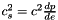from the enthapy with extra parameters (virtual function implemented in the derived classes). More...

const char * get_name () const
Returns the EOS name. More...

void set_name (const char *name_i)
Sets the EOS name. More...

Cmp nbar_ent (const Cmp &ent, int nzet, int l_min=0, Param *par=0x0) const
Computes the baryon density field from the log-enthalpy field and extra parameters. More...

Scalar nbar_ent (const Scalar &ent, int nzet, int l_min=0, Param *par=0x0) const
Computes the baryon density field from the log-enthalpy field and extra parameters. More...

Cmp ener_ent (const Cmp &ent, int nzet, int l_min=0, Param *par=0x0) const
Computes the total energy density from the log-enthalpy and extra parameters. More...

Scalar ener_ent (const Scalar &ent, int nzet, int l_min=0, Param *par=0x0) const
Computes the total energy density from the log-enthalpy and extra parameters. More...

Cmp press_ent (const Cmp &ent, int nzet, int l_min=0, Param *par=0x0) const
Computes the pressure from the log-enthalpy and extra parameters. More...

Scalar press_ent (const Scalar &ent, int nzet, int l_min=0, Param *par=0x0) const
Computes the pressure from the log-enthalpy and extra parameters. More...

Cmp der_nbar_ent (const Cmp &ent, int nzet, int l_min=0, Param *par=0x0) const
Computes the logarithmic derivativefrom the log-enthalpy and extra parameters. More...

Scalar der_nbar_ent (const Scalar &ent, int nzet, int l_min=0, Param *par=0x0) const
Computes the logarithmic derivativefrom the log-enthalpy and extra parameters. More...

Cmp der_ener_ent (const Cmp &ent, int nzet, int l_min=0, Param *par=0x0) const
Computes the logarithmic derivativefrom the log-enthalpy and extra parameters. More...

Scalar der_ener_ent (const Scalar &ent, int nzet, int l_min=0, Param *par=0x0) const
Computes the logarithmic derivativefrom the log-enthalpy and extra parameters. More...

Cmp der_press_ent (const Cmp &ent, int nzet, int l_min=0, Param *par=0x0) const
Computes the logarithmic derivativefrom the log-enthalpy and extra parameters. More...

Scalar der_press_ent (const Scalar &ent, int nzet, int l_min=0, Param *par=0x0) const
Computes the logarithmic derivativefrom the log-enthalpy and extra parameters. More...

Scalar csound_square_ent (const Scalar &ent, int nzet, int l_min=0, Param *par=0x0) const
Computes the sound speed squaredfrom the enthalpy with extra parameters. More...

## Static Public Member Functions

static Eoseos_from_file (FILE *)
Construction of an EOS from a binary file. More...

static Eoseos_from_file (ifstream &)
Construction of an EOS from a formatted file. More...

## Protected Member Functions

Eos_incomp (FILE *)
Constructor from a binary file (created by the function sauve(FILE*) ). More...

Eos_incomp (ifstream &)
Constructor from a formatted file. More...

virtual ostream & operator>> (ostream &) const
Operator >> More...

void calcule (const Cmp &thermo, int nzet, int l_min, double(Eos::*fait)(double, const Param *) const, Param *par, Cmp &resu) const
General computational method for Cmp 's. More...

void calcule (const Scalar &thermo, int nzet, int l_min, double(Eos::*fait)(double, const Param *) const, Param *par, Scalar &resu) const
General computational method for Scalar 's. More...

## Protected Attributes

double rho0
Constant density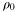. More...

double ent0
Log-enthalpy threshold for setting the energy density to a non zero value (should be negative). More...

char name 
EOS name. More...

## Friends

EosEos::eos_from_file (FILE *)
The construction functions from a file. More...

EosEos::eos_from_file (ifstream &)

## Detailed Description

Equation of state of incompressible matter (relativistic case).

This equation of state (EOS) corresponds to a constant density matter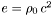. ()

Definition at line 1632 of file eos.h.

## ◆ Eos_incomp() [1/5]

 Lorene::Eos_incomp::Eos_incomp ( double rho_c )

Standard constructor.

The log-enthalpy threshold for non-zero density is set -1.e-6.

Parameters
 rho_c constant density[unit: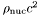], where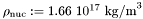Definition at line 102 of file eos_incomp.C.

## ◆ Eos_incomp() [2/5]

 Lorene::Eos_incomp::Eos_incomp ( double rho_c, double ent_c )

Standard constructor with log-enthalpy threshold.

Parameters
 rho_c constant density[unit:], whereent_c log-enthalpy threshold for non-zero density [unit: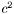] : the energy density is set to rho_c} for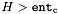.

Definition at line 108 of file eos_incomp.C.

## ◆ Eos_incomp() [3/5]

 Lorene::Eos_incomp::Eos_incomp ( const Eos_incomp & eosi )

Copy constructor.

Definition at line 118 of file eos_incomp.C.

## ◆ Eos_incomp() [4/5]

 Lorene::Eos_incomp::Eos_incomp ( FILE * fich )
protected

Constructor from a binary file (created by the function sauve(FILE*) ).

This constructor is protected because any EOS construction from a binary file must be done via the function Eos::eos_from_file(FILE*) .

Definition at line 125 of file eos_incomp.C.

## ◆ Eos_incomp() [5/5]

 Lorene::Eos_incomp::Eos_incomp ( ifstream & fich )
protected

Constructor from a formatted file.

This constructor is protected because any EOS construction from a formatted file must be done via the function Eos::eos_from_file(ifstream&) .

Definition at line 135 of file eos_incomp.C.

References ent0, and rho0.

## ◆ ~Eos_incomp()

 Lorene::Eos_incomp::~Eos_incomp ( )
virtual

Destructor.

Definition at line 148 of file eos_incomp.C.

## ◆ calcule() [1/2]

 void Lorene::Eos::calcule ( const Cmp & thermo, int nzet, int l_min, double(Eos::*)(double, const Param *) const fait, Param * par, Cmp & resu ) const
protectedinherited

General computational method for Cmp 's.

Parameters
 thermo [input] thermodynamical quantity (for instance the enthalpy field)from which the thermodynamical quantity resu is to be computed. nzet [input] number of domains where resu is to be computed. l_min [input] index of the innermost domain is which resu is to be computed [default value: 0]; resu is computed only in domains whose indices are in [l_min,l_min+nzet-1] . In the other domains, it is set to zero. fait [input] pointer on the member function of class Eos which performs the pointwise calculation. par possible extra parameters of the EOS resu [output] result of the computation.

Definition at line 213 of file eos.C.

References Lorene::Cmp::get_etat().

## ◆ calcule() [2/2]

 void Lorene::Eos::calcule ( const Scalar & thermo, int nzet, int l_min, double(Eos::*)(double, const Param *) const fait, Param * par, Scalar & resu ) const
protectedinherited

General computational method for Scalar 's.

Parameters
 thermo [input] thermodynamical quantity (for instance the enthalpy field)from which the thermodynamical quantity resu is to be computed. nzet [input] number of domains where resu is to be computed. l_min [input] index of the innermost domain is which resu is to be computed [default value: 0]; resu is computed only in domains whose indices are in [l_min,l_min+nzet-1] . In the other domains, it is set to zero. fait [input] pointer on the member function of class Eos which performs the pointwise calculation. par possible extra parameters of the EOS resu [output] result of the computation.

Definition at line 285 of file eos.C.

References Lorene::Scalar::get_etat().

## ◆ csound_square_ent()

 Scalar Lorene::Eos::csound_square_ent ( const Scalar & ent, int nzet, int l_min = 0, Param * par = 0x0 ) const
inherited

Computes the sound speed squaredfrom the enthalpy with extra parameters.

Parameters
 ent [input, unit: c^2] enthalpy nzet number of domains where the derivative dln(e)/dln(H) is to be computed. l_min index of the innermost domain is which the coefficient dln(n)/dln(H) is to be computed [default value: 0]; the derivative dln(e)/dln(H) is computed only in domains whose indices are in [l_min,l_min+nzet-1] . In the other domains, it is set to zero. par possible extra parameters of the EOS
Returns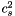[unit: c^2]

Definition at line 499 of file eos.C.

## ◆ csound_square_ent_p()

 virtual double Lorene::Eos_incomp::csound_square_ent_p ( double , const Param * ) const
inlinevirtual

Computes the sound speed squaredfrom the enthapy with extra parameters (virtual function implemented in the derived classes).

Parameters
 ent [input, unit: c^2] enthalpy par possible extra parameters of the EOS
Returns[unit: c^2]

Implements Lorene::Eos.

Reimplemented in Lorene::Eos_incomp_newt.

Definition at line 1797 of file eos.h.

References Lorene::c_est_pas_fait().

## ◆ der_ener_ent() [1/2]

 Cmp Lorene::Eos::der_ener_ent ( const Cmp & ent, int nzet, int l_min = 0, Param * par = 0x0 ) const
inherited

Computes the logarithmic derivativefrom the log-enthalpy and extra parameters.

Parameters
 ent [input, unit:] log-enthalpy H defined by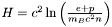, where e is the (total) energy density, p the pressure, n the baryon density, and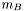the baryon mass nzet number of domains where the derivative dln(e)/dln(H) is to be computed. l_min index of the innermost domain is which the coefficient dln(n)/dln(H) is to be computed [default value: 0]; the derivative dln(e)/dln(H) is computed only in domains whose indices are in [l_min,l_min+nzet-1] . In the other domains, it is set to zero. par possible extra parameters of the EOS
Returns
dln(e)/dln(H)

Definition at line 454 of file eos.C.

## ◆ der_ener_ent() [2/2]

 Scalar Lorene::Eos::der_ener_ent ( const Scalar & ent, int nzet, int l_min = 0, Param * par = 0x0 ) const
inherited

Computes the logarithmic derivativefrom the log-enthalpy and extra parameters.

Parameters
 ent [input, unit:] log-enthalpy H defined by, where e is the (total) energy density, p the pressure, n the baryon density, andthe baryon mass nzet number of domains where the derivative dln(e)/dln(H) is to be computed. l_min index of the innermost domain is which the coefficient dln(n)/dln(H) is to be computed [default value: 0]; the derivative dln(e)/dln(H) is computed only in domains whose indices are in [l_min,l_min+nzet-1] . In the other domains, it is set to zero. par possible extra parameters of the EOS
Returns
dln(e)/dln(H)

Definition at line 464 of file eos.C.

## ◆ der_ener_ent_p()

 double Lorene::Eos_incomp::der_ener_ent_p ( double ent, const Param * par = 0x0 ) const
virtual

Computes the logarithmic derivativefrom the log-enthalpy.

Parameters
 ent [input, unit:] log-enthalpy H
Returns
dln(e)/dln(H)

Implements Lorene::Eos.

Reimplemented in Lorene::Eos_incomp_newt.

Definition at line 300 of file eos_incomp.C.

References ent0.

## ◆ der_nbar_ent() [1/2]

 Cmp Lorene::Eos::der_nbar_ent ( const Cmp & ent, int nzet, int l_min = 0, Param * par = 0x0 ) const
inherited

Computes the logarithmic derivativefrom the log-enthalpy and extra parameters.

Parameters
 ent [input, unit:] log-enthalpy H defined by, where e is the (total) energy density, p the pressure, n the baryon density, andthe baryon mass nzet number of domains where the derivative dln(n)/dln(H) is to be computed. l_min index of the innermost domain is which the coefficient dln(n)/dln(H) is to be computed [default value: 0]; the derivative dln(n)/dln(H) is computed only in domains whose indices are in [l_min,l_min+nzet-1] . In the other domains, it is set to zero. par possible extra parameters of the EOS
Returns
dln(n)/dln(H)

Definition at line 431 of file eos.C.

## ◆ der_nbar_ent() [2/2]

 Scalar Lorene::Eos::der_nbar_ent ( const Scalar & ent, int nzet, int l_min = 0, Param * par = 0x0 ) const
inherited

Computes the logarithmic derivativefrom the log-enthalpy and extra parameters.

Parameters
 ent [input, unit:] log-enthalpy H defined by, where e is the (total) energy density, p the pressure, n the baryon density, andthe baryon mass nzet number of domains where the derivative dln(n)/dln(H) is to be computed. l_min index of the innermost domain is which the coefficient dln(n)/dln(H) is to be computed [default value: 0]; the derivative dln(n)/dln(H) is computed only in domains whose indices are in [l_min,l_min+nzet-1] . In the other domains, it is set to zero. par possible extra parameters of the EOS
Returns
dln(n)/dln(H)

Definition at line 441 of file eos.C.

## ◆ der_nbar_ent_p()

 double Lorene::Eos_incomp::der_nbar_ent_p ( double ent, const Param * par = 0x0 ) const
virtual

Computes the logarithmic derivativefrom the log-enthalpy.

Parameters
 ent [input, unit:] log-enthalpy H
Returns
dln(n)/dln(H)

Implements Lorene::Eos.

Reimplemented in Lorene::Eos_incomp_newt.

Definition at line 286 of file eos_incomp.C.

References ent0.

## ◆ der_press_ent() [1/2]

 Cmp Lorene::Eos::der_press_ent ( const Cmp & ent, int nzet, int l_min = 0, Param * par = 0x0 ) const
inherited

Computes the logarithmic derivativefrom the log-enthalpy and extra parameters.

Parameters
 ent [input, unit:] log-enthalpy H defined by, where e is the (total) energy density, p the pressure, n the baryon density, andthe baryon mass nzet number of domains where the derivative dln(p)/dln(H) is to be computed. par possible extra parameters of the EOS l_min index of the innermost domain is which the coefficient dln(n)/dln(H) is to be computed [default value: 0]; the derivative dln(p)/dln(H) is computed only in domains whose indices are in [l_min,l_min+nzet-1] . In the other domains, it is set to zero.
Returns
dln(p)/dln(H)

Definition at line 476 of file eos.C.

## ◆ der_press_ent() [2/2]

 Scalar Lorene::Eos::der_press_ent ( const Scalar & ent, int nzet, int l_min = 0, Param * par = 0x0 ) const
inherited

Computes the logarithmic derivativefrom the log-enthalpy and extra parameters.

Parameters
 ent [input, unit:] log-enthalpy H defined by, where e is the (total) energy density, p the pressure, n the baryon density, andthe baryon mass nzet number of domains where the derivative dln(p)/dln(H) is to be computed. par possible extra parameters of the EOS l_min index of the innermost domain is which the coefficient dln(n)/dln(H) is to be computed [default value: 0]; the derivative dln(p)/dln(H) is computed only in domains whose indices are in [l_min,l_min+nzet-1] . In the other domains, it is set to zero.
Returns
dln(p)/dln(H)

Definition at line 486 of file eos.C.

## ◆ der_press_ent_p()

 double Lorene::Eos_incomp::der_press_ent_p ( double ent, const Param * par = 0x0 ) const
virtual

Computes the logarithmic derivativefrom the log-enthalpy.

Parameters
 ent [input, unit:] log-enthalpy H
Returns
dln(p)/dln(H)

Implements Lorene::Eos.

Reimplemented in Lorene::Eos_incomp_newt.

Definition at line 314 of file eos_incomp.C.

References ent0, and Lorene::exp().

## ◆ ener_ent() [1/2]

 Cmp Lorene::Eos::ener_ent ( const Cmp & ent, int nzet, int l_min = 0, Param * par = 0x0 ) const
inherited

Computes the total energy density from the log-enthalpy and extra parameters.

Parameters
 ent [input, unit:] log-enthalpy H defined by, where e is the (total) energy density, p the pressure, n the baryon density, andthe baryon mass nzet number of domains where the energy density is to be computed. l_min index of the innermost domain is which the energy density is to be computed [default value: 0]; the energy density is computed only in domains whose indices are in [l_min,l_min+nzet-1] . In the other domains, it is set to zero. par possible extra parameters of the EOS
Returns
energy density [unit:], whereDefinition at line 387 of file eos.C.

References Lorene::Eos::calcule(), Lorene::Eos::ener_ent_p(), and Lorene::Cmp::get_mp().

## ◆ ener_ent() [2/2]

 Scalar Lorene::Eos::ener_ent ( const Scalar & ent, int nzet, int l_min = 0, Param * par = 0x0 ) const
inherited

Computes the total energy density from the log-enthalpy and extra parameters.

Parameters
 ent [input, unit:] log-enthalpy H defined by, where e is the (total) energy density, p the pressure, n the baryon density, andthe baryon mass nzet number of domains where the energy density is to be computed. l_min index of the innermost domain is which the energy density is to be computed [default value: 0]; the energy density is computed only in domains whose indices are in [l_min,l_min+nzet-1] . In the other domains, it is set to zero. par possible extra parameters of the EOS
Returns
energy density [unit:], whereDefinition at line 397 of file eos.C.

## ◆ ener_ent_p()

 double Lorene::Eos_incomp::ener_ent_p ( double ent, const Param * par = 0x0 ) const
virtual

Computes the total energy density from the log-enthalpy.

Parameters
 ent [input, unit:] log-enthalpy H
Returns
energy density e [unit:], whereImplements Lorene::Eos.

Reimplemented in Lorene::Eos_incomp_newt.

Definition at line 257 of file eos_incomp.C.

References ent0, and rho0.

## ◆ eos_from_file() [1/2]

 Eos * Lorene::Eos::eos_from_file ( FILE * fich )
staticinherited

Construction of an EOS from a binary file.

The file must have been created by the function sauve(FILE*) .

Definition at line 193 of file eos_from_file.C.

## ◆ eos_from_file() [2/2]

 Eos * Lorene::Eos::eos_from_file ( ifstream & fich )
staticinherited

Construction of an EOS from a formatted file.

The fist line of the file must start by the EOS number, according to the following conventions:

• 1 = relativistic polytropic EOS (class Eos_poly ).
• 2 = Newtonian polytropic EOS (class Eos_poly_newt ).
• 3 = Relativistic incompressible EOS (class Eos_incomp ).
• 4 = Newtonian incompressible EOS (class Eos_incomp_newt ).
• 5 = Strange matter (MIT Bag model)
• 6 = Strange matter (MIT Bag model) with crust
• 10 = SLy4 (Douchin & Haensel 2001)
- 11 = FPS (Friedman-Pandharipande + Skyrme)
• 12 = BPAL12 (Bombaci et al. 1995)
• 13 = AkmalPR (Akmal, Pandharipande & Ravenhall 1998)
• 14 = BBB2 (Baldo, Bombaci & Burgio 1997)
• 15 = BalbN1H1 (Balberg 2000)
• 16 = GlendNH3 (Glendenning 1985, case 3)
• 17 = Tabulated EOS in CompOSE format
• 18 = magnetized (tabulated) equation of state
• 19 = relativistic ideal Fermi gas at zero temperature (class Eos_Fermi)
• 20 = Tabulated EOS in CompOSE format corrected for thermo. consistency
• 100 = Multi-domain EOS (class MEos )
• 110 = Multi-polytropic EOS (class Eos_multi_poly )
• 120 = Fitted SLy4 (Shibata 2004)
• 121 = Fitted FPS (Shibata 2004)
• 122 = Fitted AkmalPR (Taniguchi 2005)

The second line in the file should contain a name given by the user to the EOS. The following lines should contain the EOS parameters (one parameter per line), in the same order than in the class declaration.

Definition at line 345 of file eos_from_file.C.

## ◆ get_name()

 const char * Lorene::Eos::get_name ( ) const
inherited

Returns the EOS name.

Definition at line 179 of file eos.C.

References Lorene::Eos::name.

## ◆ identify()

 int Lorene::Eos_incomp::identify ( ) const
virtual

Returns a number to identify the sub-classe of Eos the object belongs to.

Implements Lorene::Eos.

Reimplemented in Lorene::Eos_incomp_newt.

Definition at line 143 of file eos_from_file.C.

## ◆ nbar_ent() [1/2]

 Cmp Lorene::Eos::nbar_ent ( const Cmp & ent, int nzet, int l_min = 0, Param * par = 0x0 ) const
inherited

Computes the baryon density field from the log-enthalpy field and extra parameters.

Parameters
 ent [input, unit:] log-enthalpy H defined by, where e is the (total) energy density, p the pressure, n the baryon density, andthe baryon mass nzet number of domains where the baryon density is to be computed. l_min index of the innermost domain is which the baryon density is to be computed [default value: 0]; the baryon density is computed only in domains whose indices are in [l_min,l_min+nzet-1] . In the other domains, it is set to zero. par possible extra parameters of the EOS
Returns
baryon density [unit: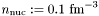]

Definition at line 362 of file eos.C.

References Lorene::Eos::calcule(), Lorene::Cmp::get_mp(), and Lorene::Eos::nbar_ent_p().

## ◆ nbar_ent() [2/2]

 Scalar Lorene::Eos::nbar_ent ( const Scalar & ent, int nzet, int l_min = 0, Param * par = 0x0 ) const
inherited

Computes the baryon density field from the log-enthalpy field and extra parameters.

Parameters
 ent [input, unit:] log-enthalpy H defined by, where e is the (total) energy density, p the pressure, n the baryon density, andthe baryon mass nzet number of domains where the baryon density is to be computed. l_min index of the innermost domain is which the baryon density is to be computed [default value: 0]; the baryon density is computed only in domains whose indices are in [l_min,l_min+nzet-1] . In the other domains, it is set to zero. par possible extra parameters of the EOS
Returns
baryon density [unit:]

Definition at line 372 of file eos.C.

## ◆ nbar_ent_p()

 double Lorene::Eos_incomp::nbar_ent_p ( double ent, const Param * par = 0x0 ) const
virtual

Computes the baryon density from the log-enthalpy.

Parameters
 ent [input, unit:] log-enthalpy H
Returns
baryon density n [unit:]

Implements Lorene::Eos.

Reimplemented in Lorene::Eos_incomp_newt.

Definition at line 243 of file eos_incomp.C.

References ent0, and rho0.

## ◆ operator!=()

 bool Lorene::Eos_incomp::operator!= ( const Eos & eos_i ) const
virtual

Comparison operator (difference)

Implements Lorene::Eos.

Reimplemented in Lorene::Eos_incomp_newt.

Definition at line 202 of file eos_incomp.C.

References operator==().

## ◆ operator=()

 void Lorene::Eos_incomp::operator= ( const Eos_incomp & eosi )

Assignment to another Eos_incomp.

Definition at line 157 of file eos_incomp.C.

References ent0, Lorene::Eos::name, rho0, and Lorene::Eos::set_name().

## ◆ operator==()

 bool Lorene::Eos_incomp::operator== ( const Eos & eos_i ) const
virtual

Comparison operator (egality)

Implements Lorene::Eos.

Reimplemented in Lorene::Eos_incomp_newt.

Definition at line 170 of file eos_incomp.C.

References ent0, Lorene::Eos::identify(), identify(), and rho0.

## ◆ operator>>()

 ostream & Lorene::Eos_incomp::operator>> ( ostream & ost ) const
protectedvirtual

Operator >>

Implements Lorene::Eos.

Reimplemented in Lorene::Eos_incomp_newt.

Definition at line 223 of file eos_incomp.C.

References ent0, and rho0.

## ◆ press_ent() [1/2]

 Cmp Lorene::Eos::press_ent ( const Cmp & ent, int nzet, int l_min = 0, Param * par = 0x0 ) const
inherited

Computes the pressure from the log-enthalpy and extra parameters.

Parameters
 ent [input, unit:] log-enthalpy H defined by, where e is the (total) energy density, p the pressure, n the baryon density, andthe baryon mass nzet number of domains where the pressure is to be computed. l_min index of the innermost domain is which the pressure is to be computed [default value: 0]; the pressure is computed only in domains whose indices are in [l_min,l_min+nzet-1] . In the other domains, it is set to zero. par possible extra parameters of the EOS
Returns
pressure [unit:], whereDefinition at line 409 of file eos.C.

## ◆ press_ent() [2/2]

 Scalar Lorene::Eos::press_ent ( const Scalar & ent, int nzet, int l_min = 0, Param * par = 0x0 ) const
inherited

Computes the pressure from the log-enthalpy and extra parameters.

Parameters
 ent [input, unit:] log-enthalpy H defined by, where e is the (total) energy density, p the pressure, n the baryon density, andthe baryon mass nzet number of domains where the pressure is to be computed. l_min index of the innermost domain is which the pressure is to be computed [default value: 0]; the pressure is computed only in domains whose indices are in [l_min,l_min+nzet-1] . In the other domains, it is set to zero. par possible extra parameters of the EOS
Returns
pressure [unit:], whereDefinition at line 419 of file eos.C.

## ◆ press_ent_p()

 double Lorene::Eos_incomp::press_ent_p ( double ent, const Param * par = 0x0 ) const
virtual

Computes the pressure from the log-enthalpy.

Parameters
 ent [input, unit:] log-enthalpy H
Returns
pressure p [unit:], whereImplements Lorene::Eos.

Reimplemented in Lorene::Eos_incomp_newt.

Definition at line 271 of file eos_incomp.C.

References ent0, Lorene::exp(), and rho0.

## ◆ sauve()

 void Lorene::Eos_incomp::sauve ( FILE * fich ) const
virtual

Save in a file.

Reimplemented from Lorene::Eos.

Reimplemented in Lorene::Eos_incomp_newt.

Definition at line 214 of file eos_incomp.C.

References ent0, Lorene::fwrite_be(), rho0, and Lorene::Eos::sauve().

## ◆ set_name()

 void Lorene::Eos::set_name ( const char * name_i )
inherited

Sets the EOS name.

Definition at line 173 of file eos.C.

References Lorene::Eos::name.

## ◆ Eos::eos_from_file

 Eos* Eos::eos_from_file ( FILE * )
friend

The construction functions from a file.

## ◆ ent0

 double Lorene::Eos_incomp::ent0
protected

Log-enthalpy threshold for setting the energy density to a non zero value (should be negative).

Definition at line 1644 of file eos.h.

## ◆ name

 char Lorene::Eos::name
protectedinherited

EOS name.

Definition at line 212 of file eos.h.

## ◆ rho0

 double Lorene::Eos_incomp::rho0
protected

Constant density.

Definition at line 1639 of file eos.h.

The documentation for this class was generated from the following files: